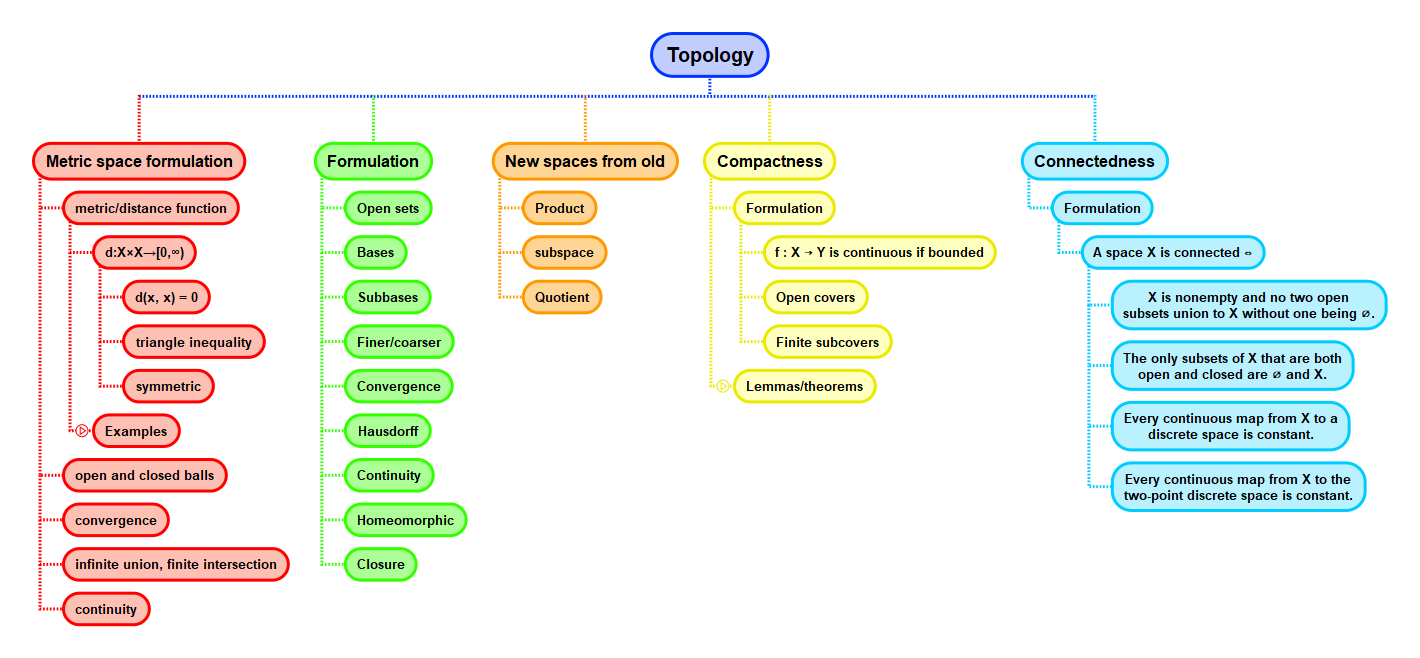Show Question
Math and science::Topology

# Compactness. Examples

• The collection of sets $$\{(n - 1, n + 1) : n \in \mathbb{Z} \}$$ form an open cover of $$\mathbb{R}$$. This cover has no finite subcover. So $$\mathbb{R}$$ is not compact.
• Divide the interval $$[0, 1]$$ like so: $$U_0 = [0, \frac{1}{2}]$$, $$U_n = (2^{-n}, 1]$$ for $$n \ge 1$$, then form the cover $$(U_n)_{n \ge 0}$$. This is an open cover, and has many finite subcovers (e.g. ([0, 1/2), (1/2, 1], (1/4, 1]). So we can say that $$[0, 1]$$ is .We can't say anything. We would need to prove that all open covers have a finite subcover to prove compactness.
• The compact subspaces of $$\mathbb{R}^n$$ are the closed bounded subsets.
• Any indiscrete space is compact.
• Any finite space is compact.
• A discrete space is compact iff it is finite.
• In a normed vector space $$V$$, the closed unit ball is compact iff $$V$$ is finite-dimensional.

The last example alludes to the idea that compactness is a kind of finiteness condition:

 sets finite sets vector spaces finite dimensional vector spaces topological spaces compact topological spaces

## Context